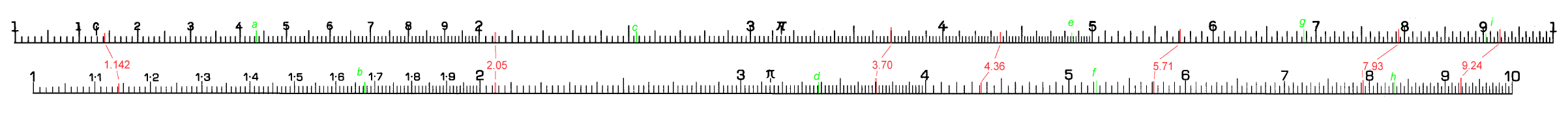This page shows how to interpret the numbering on the scales of a slide rule as the uneven separation between the graduations means it is not straightforward.

The image below (left bigger than the screen to maintain the detail) shows typical scales from two different slide rules. In the lower image, the graduations between 1 and 2 are marked 1.1 to 1.9, in the upper image they are marked 1 to 9. Although at first the lower arrangement might seem more sensible, with a little practice either arrangement will become familiar. You might also find other variations, for example some rules mark the graduation for 2.5.

One concept a user needs to be familiar with is that of "significant figures". The number for p (the ratio of the circumference to the diameter of a circle) can be expressed as 3.141592654 to 10 significant figures; that is the number is given with 10 separate digits. If it was given as 3.14, it would be represented as having 3 significant figures. On a slide rule you can normally work to a precision of 3, and sometimes 4, significant figures.

The lettering and lines in red show pairs of values on the two types of rule. The first number (1.142) shows that at the left hand end of a rule it is usually possible to estimate a value to a precision of a 4 significant figures. Though in this example  (where the red line is thicker than the marking) it would be difficult to decide whether the value was 1.142 or 1.141. As we move along the rule to the next number (2.05) we already see that three significant figures are all the we can read with accuracy. The next number is included to show a reading where the value corresponds to one of the marked graduations. Another difference between the rules is demonstrated by the number 4.36, which in the upper scale corresponds to a marked graduation but in the lower scale has to be estimated. As we move further to the right was see that the space between graduations becomes smaller and more and more care is needed in the estimation of the number.

It would be a good idea to look at all the figures in red and make sure the numbers and graduations make sense to you.Now you should make a note of what you think is the value of each of the lines marked in green. The answers are given here. Do not worry too much if your estimate of the final figure is different by 1.